Showing posts with label Grace. Show all posts
Showing posts with label Grace. Show all posts

## Friday, December 14, 2012

### It's Final Endgame for GRAIL's Twin Spacecraft

The mountain where the two spacecraft will make contact is located near a crater named Goldschmidt. Both spacecraft have been flying in formation around the moon since Jan. 1, 2012. They were named by elementary school students in Bozeman, Mont., who won a contest. The first probe to reach the moon, Ebb, also will be the first to go down, at 2:28:40 p.m. PST. Flow will follow Ebb about 20 seconds later. See: NASA Probes Prepare for Mission-Ending Moon ImpactGRAIL's Final Resting Spot These maps of Earth's moon highlight the region where the twin spacecraft of NASA's Gravity Recovery and Interior Laboratory (GRAIL) mission will impact on Dec. 17, marking the end of its successful endeavor to map the moon's gravity. The two washing-machine-sized spacecraft, named Ebb and Flow, will impact at an unnamed mountain near the moon's North Pole. These maps are from NASA's Lunar Reconnaissance Orbiter. Credit: NASA/GSFC

## Saturday, December 08, 2012

### GRAIL's Gravity Tour of the Moon

Embedded video from
NASA Jet Propulsion Laboratory California Institute of Technology
This movie shows the variations in the lunar gravity field as measured by NASA's Gravity Recovery and Interior Laboratory (GRAIL) during the primary mapping mission from March to May 2012.

## Thursday, October 25, 2012

### Gravimetry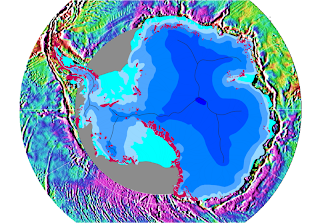Gravity map of the Southern Ocean around the Antarctic continent This gravity field was computed from sea-surface height measurements collected by the US Navy GEOSAT altimeter between March, 1985, and January, 1990. The high density GEOSAT Geodetic Mission data that lie south of 30 deg. S were declassified by the Navy in May of 1992 and contribute most of the fine-scale gravity information. The Antarctic continent itself is shaded in blue depending on the thickness of the ice sheet (blue shades in steps of 1000 m); light blue is shelf ice; gray lines are the major ice devides; pink spots are parts of the continent which are not covered by ice; gray areas have no data.

Gravimetry is the measurement of the strength of a gravitational field. Gravimetry may be used when either the magnitude of gravitational field or the properties of matter responsible for its creation are of interest. The term gravimetry or gravimetric is also used in chemistry to define a class of analytical procedures, called gravimetric analysis relying upon weighing a sample of material.

Atom interferometry: In light-pulse atom interferometers, atomic matter waves are split and recombined using pulses of laser light. The splitting occurs because when an atom interacts with the photons of a laser beam, it exchanges the momentum of a number of photons. The atom may thus continue on either of two spatially separate paths, the interferometer arms. When the paths are recombined, the probability that the atom is found depends upon the phase difference between them, which determines whether the matter waves will add or cancel. This phase is shifted by the atom’s coupling to electromagnetic fields, gravity, inertial forces, and other influences. By selecting the geometry of the interferometer, the atomic species, and its quantum state, one can maximize the wanted influence and minimize others. Advances in the control of the quantum state of atoms and photons have led to an extraordinary sensitivity and accuracy.

See:

## Sunday, July 15, 2012

### Space Geodesy

Project manager Stephen Merkowitz talks about his work with NASA's Space Geodesy Project, including a brief overview of the four fundamental techniques of space geodesy: GPS, VLBI, SLR, and DORIS.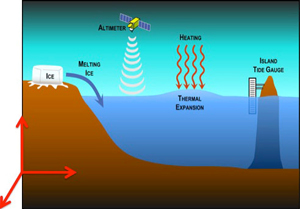Space Geodesy provides positioning, navigation, and timing reference systems and Earth system observations
Geodesy is the science of the Earth’s shape, gravity and rotation, including their evolution in time. A number of different techniques are used to observe the geodetic properties of the Earth including the space-geodetic techniques of Very Long Baseline Interferometry (VLBI), Satellite Laser Ranging (SLR), Global Navigation Satellite Systems (GNSS) like the US Global Positioning System (GPS), and the French Doppler Orbitography and Radio-Positioning by Integrated Satellite (DORIS) system. These space-geodetic observations also provide the basis for the reference frame that is needed in order to assign coordinates to points and objects and thereby determine how those points and objects move over time. See SGP Science

## Tuesday, March 06, 2012

### Geodesy and geophysicsMean Gravity Field

Anomalies by definition would require that we understand something about our selves that we did not know before, in that the whole history of you, is a large synopsis of everything that thinks and breath? Imagine indeed that such a vast resource could defined you as in some book to know that what would come next would be the unfolding of what you have been to what you shall become.

So I veered away from this act of who you are, toward a question of our relationship with understanding the world around us. What can exist in nature as some anomaly is really our inability to describe something in nature that awes us and had never gone deeper then then on the surface observance of who we are and where we live.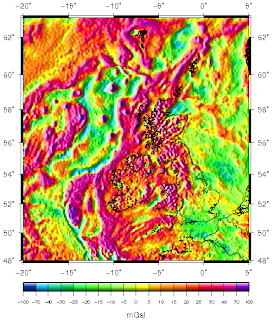Map of free-air gravity anomalies around Britain and Ireland
Variations in the strength of gravity occur from place to place according to the density distribution of the rocks beneath the surface. Such gravity anomalies have been mapped across the British Isles and the surrounding seas and they reveal aspects of these islands’ geological structure.(Bouguer) gravity anomaly map of the state of New Jersey (USGS)
The Bouguer anomalies usually are negative in the mountains because of isostasy: the rock density of their roots is lower, compared with the surrounding earth's mantle. Typical anomalies in the Central Alps are −150 milligals (−1.5 mm/s²). Rather local anomalies are used in applied geophysics: if they are positive, this may indicate metallic ores. At scales between entire mountain ranges and ore bodies, Bouguer anomalies may indicate rock types. For example, the northeast-southwest trending high across central New Jersey (see figure) represents a graben of Triassic age largely filled with dense basalts. Salt domes are typically expressed in gravity maps as lows, because salt has a low density compared to the rocks the dome intrudes. Anomalies can help to distinguish sedimentary basins whose fill differs in density from that of the surrounding region - see Gravity Anomalies of Britain and Ireland for example.## Bouguer Anomaly Map of Belgium and Surrounding areas

The anomalies are calculated using a uniform Bouguer reduction density of 2.67 gr/cm3 the grid was obtained by Krigging, cell size : 5 km, search radius : 30 km. The French data are copyrighted by BRGM (France).

While the examples above help to shed light on how we can perceive earth in a way that we are not accustomed too, this idea of gravity is important to understand the way in which gravitationally we my look at the world/earth. In our early years abstractly the idea of curvature was something that did not make sense until one moved beyond the Euclidean lines of understanding to a Non Euclidean view?

To that point we did not understand what the earth look like in its pearl form in space without having left the confines of earth?

I want you too look at space as well to understand what may be conceivable even though we may talk about an anomaly in nature that to this point we did not necessarily understand until we were presented with the examples as to confront and require an explanation. So you have the earth and space to contend with here.### LTool

So we graduate with the understanding with how we have seen the earth in observatory implicitness to have it detailed in a more "ugly definition of its composed parts?" To me it's not really that ugly at all,  although as you venture to take in the color representation of the component parts of our earth's geological structure,  you learn to understand the concreteness of our definitions.

## Sunday, January 01, 2012

### Grail: Gravity Recovery and Interior Laboratory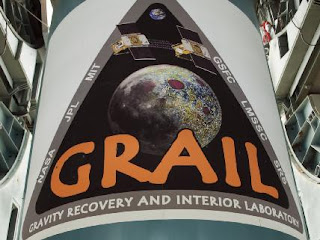### GRAIL Spacecraft Logo

NASA's Gravity Recovery and Interior Laboratory, or GRAIL, spacecraft logo is emblazoned on the first stage of a United Launch Alliance Delta II launch vehicle, now secured in the gantry at Cape Canaveral Air Force Station's Space Launch Complex 17B.

Image credit: NASA/Jim Grossmann

Mission Overview

The GRAIL mission will place two spacecraft into the same orbit around the Moon. As they fly over areas of greater and lesser gravity, caused both by visible features such as mountains and craters and by masses hidden beneath the lunar surface, they will move slightly toward and away from each other. An instrument aboard each spacecraft will measure the changes in their relative velocity very precisely, and scientists will translate this information into a high-resolution map of the Moon's gravitational field.

This gravity-measuring technique is essentially the same as that of the Gravity Recovery And Climate Experiment (GRACE), which has been mapping Earth's gravity since 2002. See: Grail: Gravity Recovery and Interior LaboratoryMean Gravity Field

Who of us could forget what the earth looks like after it has been mapped.

On planet Earth, we tend to think of the gravitational effect as being the same no matter where we are on the planet. We certainly don't see variations anywhere near as dramatic as those between the Earth and the Moon. But the truth is, the Earth's topography is highly variable with mountains, valleys, plains, and deep ocean trenches. As a consequence of this variable topography, the density of Earth's surface varies. These fluctuations in density cause slight variations in the gravity field, which, remarkably, GRACE can detect from space.

Our views in terms of the gravity field becomes part and parcel of our assessment as we venture out into space. So why not the Moon.

Image Credit: NASA/Goddard

Early assessment of Clementine along with LCROSS paints a interesting feature of our Moon as we look to understand the matter constituent makeup of the moon,  along with what it's gravity field.

Here at Dialogos of Eide I am concerned about this relationship. Such mapping not only becomes useful in the determination of the gravity field but it also heightens the understanding of relating to the elemental.

Future moon missions will need to understand the elemental makeup (while quantum gravity and relativity have not been joined experimentally) in order to use the elements to assist the colony in providing the tools necessary for it's survival there. With a Treaty established such claims to the moon become a societal move beyond earth's domain and truly moves us to civilization that will habitat the stars.

Part of this move into the cosmos will be the need to understand "something spiritual about ourselves and while ethereal in it's assessment this relationship to gravity."  It is also necessary to go "even deeper" to understand our ability to manipulate the force of gravity as a product of the mechanism of the Higg's field as we move through our own psychological underpinnings with the way in which we choose to live. (I know we have yet to proof this connection).

I give some inkling with the four links below. This is my assessment of the relationship toward "my gravity"  as I choose to live in the world of reality.

## Thursday, December 09, 2010

### Time Dilation

....... is a phenomenon (or two phenomena, as mentioned below) described by the theory of relativity. It can be illustrated by supposing that two observers are in motion relative to each other, or differently situated with regard to nearby gravitational masses. They each carry a clock of identical construction and function. Then, the point of view of each observer will generally be that the other observer's clock is in error (has changed its rate).

Both causes (distance to gravitational mass and relative speed) can operate together.

## Overview

Time dilation can arise from:
1. the relative velocity of motion between two observers, or
2. the difference in their distance from a gravitational mass.

### Relative velocity time dilation

When two observers are in relative uniform motion and far away from any gravitational mass, the point of view of each will be that the other's (moving) clock is ticking at a slower rate than the local clock. The faster the relative velocity, the greater the magnitude of time dilation. This case is sometimes called special relativistic time dilation. It is often interpreted as time "slowing down" for the other (moving) clock. But that is only true from the physical point of view of the local observer, and of others at relative rest (i.e. in the local observer's frame of reference). The point of view of the other observer will be that again the local clock (this time the other clock) is correct and it is the distant moving one that is slow. From a local perspective, time registered by clocks that are at rest with respect to the local frame of reference (and far from any gravitational mass) always appears to pass at the same rate.

### Gravitational time dilation

There is another case of time dilation, where both observers are differently situated in their distance from a significant gravitational mass, such as (for terrestrial observers) the Earth or the Sun. One may suppose for simplicity that the observers are at relative rest (which is not the case of two observers both rotating with the Earth—an extra factor described below). In the simplified case, the general theory of relativity describes how, for both observers, the clock that is closer to the gravitational mass, i.e. deeper in its "gravity well", appears to go slower than the clock that is more distant from the mass (or higher in altitude away from the center of the gravitational mass). That does not mean that the two observers fully agree: each still makes the local clock to be correct; the observer more distant from the mass (higher in altitude) measures the other clock (closer to the mass, lower in altitude) to be slower than the local correct rate, and the observer situated closer to the mass (lower in altitude) measures the other clock (farther from the mass, higher in altitude) to be faster than the local correct rate. They agree at least that the clock nearer the mass is slower in rate and on the ratio of the difference.

### Time dilation: special vs. general theories of relativity

In Albert Einstein's theories of relativity, time dilation in these two circumstances can be summarized:
• In special relativity (or, hypothetically far from all gravitational mass), clocks that are moving with respect to an inertial system of observation are measured to be running slower. This effect is described precisely by the Lorentz transformation.
Thus, in special relativity, the time dilation effect is reciprocal: as observed from the point of view of either of two clocks which are in motion with respect to each other, it will be the other clock that is time dilated. (This presumes that the relative motion of both parties is uniform; that is, they do not accelerate with respect to one another during the course of the observations.)

In contrast, gravitational time dilation (as treated in general relativity) is not reciprocal: an observer at the top of a tower will observe that clocks at ground level tick slower, and observers on the ground will agree about that, i.e. about the direction and the ratio of the difference. There is not full agreement, all the observers make their own local clocks out to be correct, but the direction and ratio of gravitational time dilation is agreed by all observers, independent of their altitude.

## Simple inference of time dilation due to relative velocity

Time dilation can be inferred from the observed fact of the constancy of the speed of light in all reference frames.    

This constancy of the speed of light means, counter to intuition, that speeds of material objects and light are not additive. It is not possible to make the speed of light appear faster by approaching at speed towards the material source that is emitting light. It is not possible to make the speed of light appear slower by receding from the source at speed. From one point of view, it is the implications of this unexpected constancy that take away from constancies expected elsewhere.

Consider a simple clock consisting of two mirrors A and B, between which a light pulse is bouncing. The separation of the mirrors is L and the clock ticks once each time it hits a given mirror.
In the frame where the clock is at rest (diagram at right), the light pulse traces out a path of length 2L and the period of the clock is 2L divided by the speed of light: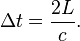$\Delta t = \frac{2 L}{c}.$
From the frame of reference of a moving observer traveling at the speed v (diagram at lower right), the light pulse traces out a longer, angled path. The second postulate of special relativity states that the speed of light is constant in all frames, which implies a lengthening of the period of this clock from the moving observer's perspective. That is to say, in a frame moving relative to the clock, the clock appears to be running more slowly. Straightforward application of the Pythagorean theorem leads to the well-known prediction of special relativity:

The total time for the light pulse to trace its path is given by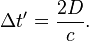$\Delta t' = \frac{2 D}{c}.$
The length of the half path can be calculated as a function of known quantities as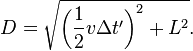$D = \sqrt{\left (\frac{1}{2}v \Delta t'\right )^2+L^2}.$
Substituting D from this equation into the previous and solving for Δt' gives: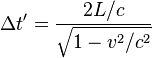$\Delta t' = \frac{2L/c}{\sqrt{1-v^2/c^2}}$
and thus, with the definition of Δt: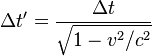$\Delta t' = \frac{\Delta t}{\sqrt{1-v^2/c^2}}$
which expresses the fact that for the moving observer the period of the clock is longer than in the frame of the clock itself.

## Time dilation due to relative velocity symmetric between observers

Common sense would dictate that if time passage has slowed for a moving object, the moving object would observe the external world to be correspondingly "sped up". Counterintuitively, special relativity predicts the opposite.

A similar oddity occurs in everyday life. If Sam sees Abigail at a distance she appears small to him and at the same time Sam appears small to Abigail. Being very familiar with the effects of perspective, we see no mystery or a hint of a paradox in this situation.

One is accustomed to the notion of relativity with respect to distance: the distance from Los Angeles to New York is by convention the same as the distance from New York to Los Angeles. On the other hand, when speeds are considered, one thinks of an object as "actually" moving, overlooking that its motion is always relative to something else — to the stars, the ground or to oneself. If one object is moving with respect to another, the latter is moving with respect to the former and with equal relative speed.

In the special theory of relativity, a moving clock is found to be ticking slowly with respect to the observer's clock. If Sam and Abigail are on different trains in near-lightspeed relative motion, Sam measures (by all methods of measurement) clocks on Abigail's train to be running slowly and similarly, Abigail measures clocks on Sam's train to be running slowly.

Note that in all such attempts to establish "synchronization" within the reference system, the question of whether something happening at one location is in fact happening simultaneously with something happening elsewhere, is of key importance. Calculations are ultimately based on determining which events are simultaneous. Furthermore, establishing simultaneity of events separated in space necessarily requires transmission of information between locations, which by itself is an indication that the speed of light will enter the determination of simultaneity.

It is a natural and legitimate question to ask how, in detail, special relativity can be self-consistent if clock A is time-dilated with respect to clock B and clock B is also time-dilated with respect to clock A. It is by challenging the assumptions built into the common notion of simultaneity that logical consistency can be restored. Simultaneity is a relationship between an observer in a particular frame of reference and a set of events. By analogy, left and right are accepted to vary with the position of the observer, because they apply to a relationship. In a similar vein, Plato explained that up and down describe a relationship to the earth and one would not fall off at the antipodes.

Within the framework of the theory and its terminology there is a relativity of simultaneity that affects how the specified events are aligned with respect to each other by observers in relative motion. Because the pairs of putatively simultaneous moments are identified differently by different observers (as illustrated in the twin paradox article), each can treat the other clock as being the slow one without relativity being self-contradictory. This can be explained in many ways, some of which follow.

### Temporal coordinate systems and clock synchronization

In Relativity, temporal coordinate systems are set up using a procedure for synchronizing clocks, discussed by Poincaré (1900) in relation to Lorentz's local time (see relativity of simultaneity). It is now usually called the Einstein synchronization procedure, since it appeared in his 1905 paper.

An observer with a clock sends a light signal out at time t1 according to his clock. At a distant event, that light signal is reflected back to, and arrives back to the observer at time t2 according to his clock. Since the light travels the same path at the same rate going both out and back for the observer in this scenario, the coordinate time of the event of the light signal being reflected for the observer tE is tE = (t1 + t2) / 2. In this way, a single observer's clock can be used to define temporal coordinates which are good anywhere in the universe.

Symmetric time dilation occurs with respect to temporal coordinate systems set up in this manner. It is an effect where another clock is being viewed as running slowly by an observer. Observers do not consider their own clock time to be time-dilated, but may find that it is observed to be time-dilated in another coordinate system.

## Overview of formulae

### Time dilation due to relative velocity

The formula for determining time dilation in special relativity is: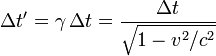$\Delta t' = \gamma \, \Delta t = \frac{\Delta t}{\sqrt{1-v^2/c^2}} \,$
where Δt is the time interval between two co-local events (i.e. happening at the same place) for an observer in some inertial frame (e.g. ticks on his clock) – this is known as the proper time, Δt ' is the time interval between those same events, as measured by another observer, inertially moving with velocity v with respect to the former observer, v is the relative velocity between the observer and the moving clock, c is the speed of light, and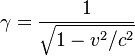$\gamma = \frac{1}{\sqrt{1-v^2/c^2}} \,$
is the Lorentz factor. Thus the duration of the clock cycle of a moving clock is found to be increased: it is measured to be "running slow". The range of such variances in ordinary life, where vc, even considering space travel, are not great enough to produce easily detectable time dilation effects and such vanishingly small effects can be safely ignored. It is only when an object approaches speeds on the order of 30,000 km/s (1/10 the speed of light) that time dilation becomes important.

Time dilation by the Lorentz factor was predicted by Joseph Larmor (1897), at least for electrons orbiting a nucleus. Thus "... individual electrons describe corresponding parts of their orbits in times shorter for the [rest] system in the ratio :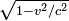$\sqrt{1 - v^2/c^2}$" (Larmor 1897). Time dilation of magnitude corresponding to this (Lorentz) factor has been experimentally confirmed, as described below.

### Time dilation due to gravitation and motion together

Astronomical time scales and the GPS system represent significant practical applications, presenting problems that call for consideration of the combined effects of mass and motion in producing time dilation.
Relativistic time dilation effects, for the solar system and the Earth, have been evaluated from the starting point of an approximation to the Schwarzschild solution to the Einstein field equations. A timelike interval dtE in this metric can be approximated, when expressed in rectangular coordinates and when truncated of higher powers in 1/c2, in the form: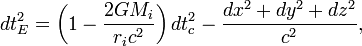$dt_E^2 = \left( 1-\frac{2GM_i}{r_i c^2} \right) dt_c^2 - \frac{dx^2+dy^2+dz^2}{c^2}, \,$
(1)
where:
dtE (expressed as a time-like interval) is a small increment forming part of an interval in the proper time tE (an interval that could be recorded on an atomic clock);
dtc is a small increment in the timelike coordinate tc ("coordinate time") of the clock's position in the chosen reference frame;
dx, dy and dz are small increments in three orthogonal space-like coordinates x, y, z of the clock's position in the chosen reference frame; and
GMi/ri represents a sum, to be designated U, of gravitational potentials due to the masses in the neighborhood, based on their distances ri from the clock. This sum of the GMi/ri is evaluated approximately, as a sum of Newtonian gravitational potentials (plus any tidal potentials considered), and is represented below as U (using the positive astronomical sign convention for gravitational potentials). The scope of the approximation may be extended to a case where U further includes effects of external masses other than the Mi, in the form of tidal gravitational potentials that prevail (due to the external masses) in a suitably small region of space around a point of the reference frame located somewhere in a gravity well due to those external masses, where the size of 'suitably small' remains to be investigated.
From this, after putting the velocity of the clock (in the coordinates of the chosen reference frame) as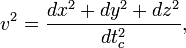$v^2=\frac{dx^2+dy^2+dz^2}{dt_c^2}, \,$
(2)
(then taking the square root and truncating after binomial expansion, neglecting terms beyond the first power in 1/c2), a relation between the rate of the proper time and the rate of the coordinate time can be obtained as the differential equation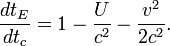$\frac{dt_E}{dt_c}= 1-\frac{U}{c^2}-\frac{v^2}{2c^2}. \,$
(3)
Equation (3) represents combined time dilations due to mass and motion, approximated to the first order in powers of 1/c2. The approximation can be applied to a number of the weak-field situations found around the Earth and in the solar-system. It can be thought of as relating the rate of proper time tE that can be measured by a clock, with the rate of a coordinate time tc.

In particular, for explanatory purposes, the time-dilation equation (3) provides a way of conceiving coordinate time, by showing that the rate of the clock would be exactly equal to the rate of the coordinate time if this "coordinate clock" could be situated
(a) hypothetically outside all relevant 'gravity wells', e.g. remote from all gravitational masses Mi, (so that U=0), and also
(b) at rest in relation to the chosen system of coordinates (so that v=0).
Equation (3) has been developed and integrated for the case where the reference frame is the solar system barycentric ('ssb') reference frame, to show the (time-varying) time dilation between the ssb coordinate time and local time at the Earth's surface: the main effects found included a mean time dilation of about 0.49 second per year (slower at the Earth's surface than for the ssb coordinate time), plus periodic modulation terms of which the largest has an annual period and an amplitude of about 1.66 millisecond.

Equation (3) has also been developed and integrated for the case of clocks at or near the Earth's surface. For clocks fixed to the rotating Earth's surface at mean sea level, regarded as a surface of the geoid, the sum ( U + v2/2 ) is a very nearly constant geopotential, and decreases with increasing height above sea level approximately as the product of the change in height and the gradient of the geopotential. This has been evaluated as a fractional increase in clock rate of about 1.1x10−13 per kilometer of height above sea level due to a decrease in combined rate of time dilation with increasing altitude. The value of dtE/dtc at height falls to be compared with the corresponding value at mean sea level. (Both values are slightly below 1, the value at height being a little larger (closer to 1) than the value at sea level.)

A fuller development of equation (3) for the near-Earth situation has been used to evaluate the combined time dilations relative to the Earth's surface experienced along the trajectories of satellites of the GPS global positioning system. The resulting values (in this case they are relativistic increases in the rate of the satellite-borne clocks, by about 38 microseconds per day) form the basis for adjustments essential for the functioning of the system.

This gravitational time dilation relationship has been used in the synchronization or correlation of atomic clocks used to implement and maintain the atomic time scale TAI, where the different clocks are located at different heights above sea level, and since 1977 have had their frequencies steered to compensate for the differences of rate with height.

In pulsar timing, the advance or retardation of the pulsar phase due to gravitational and motional time dilation is called the "Einstein Delay".

## Experimental confirmation

Time dilation has been tested a number of times. The routine work carried on in particle accelerators since the 1950s, such as those at CERN, is a continuously running test of the time dilation of special relativity. The specific experiments include:

### Velocity time dilation tests

• Ives and Stilwell (1938, 1941), "An experimental study of the rate of a moving clock", in two parts. The stated purpose of these experiments was to verify the time dilation effect, predicted by Lamor-Lorentz ether theory, due to motion through the ether using Einstein's suggestion that Doppler effect in canal rays would provide a suitable experiment. These experiments measured the Doppler shift of the radiation emitted from cathode rays, when viewed from directly in front and from directly behind. The high and low frequencies detected were not the classical values predicted.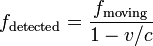$f_\mathrm{detected} = \frac{f_\mathrm{moving}}{1 - v/c}$ and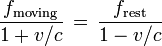$\frac{f_\mathrm{moving}}{1+v/c}\,=\, \frac{f_\mathrm{rest}}{1 - v/c}$ and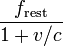$\frac{f_\mathrm{rest}}{1+v/c}$
i.e. for sources with invariant frequencies$f_\mathrm{moving}\, = f_\mathrm{rest}$ The high and low frequencies of the radiation from the moving sources were measured as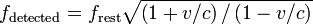$f_\mathrm{detected} = f_\mathrm{rest}\sqrt{\left(1 + v/c\right)/\left(1 - v/c\right) }$ and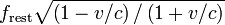$f_\mathrm{rest}\sqrt{\left(1 - v/c\right)/\left(1 + v/c\right)}$
as deduced by Einstein (1905) from the Lorentz transformation, when the source is running slow by the Lorentz factor.
• Rossi and Hall (1941) compared the population of cosmic-ray-produced muons at the top of a mountain to that observed at sea level. Although the travel time for the muons from the top of the mountain to the base is several muon half-lives, the muon sample at the base was only moderately reduced. This is explained by the time dilation attributed to their high speed relative to the experimenters. That is to say, the muons were decaying about 10 times slower than if they were at rest with respect to the experimenters.
• Hasselkamp, Mondry, and Scharmann (1979) measured the Doppler shift from a source moving at right angles to the line of sight (the transverse Doppler shift). The most general relationship between frequencies of the radiation from the moving sources is given by: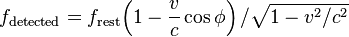$f_\mathrm{detected} = f_\mathrm{rest}{\left(1 - \frac{v}{c} \cos\phi\right)/\sqrt{1 - {v^2}/{c^2}} }$
as deduced by Einstein (1905). For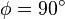$\phi = 90^\circ$ (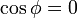$\cos\phi = 0\,$) this reduces to fdetected = frestγ. Thus there is no transverse Doppler shift, and the lower frequency of the moving source can be attributed to the time dilation effect alone.
• In 2010 time dilation was observed at speeds of less than 10 meters per second using optical atomic clocks connected by 75 meters of optical fiber.

### Gravitational time dilation tests

• Pound, Rebka in 1959 measured the very slight gravitational red shift in the frequency of light emitted at a lower height, where Earth's gravitational field is relatively more intense. The results were within 10% of the predictions of general relativity. Later Pound and Snider (in 1964) derived an even closer result of 1%. This effect is as predicted by gravitational time dilation.
• In 2010 gravitational time dilation was measured at the Earth's surface with a height difference of only one meter, using optical atomic clocks.

### Velocity and gravitational time dilation combined-effect tests

• Hafele and Keating, in 1971, flew caesium atomic clocks east and west around the Earth in commercial airliners, to compare the elapsed time against that of a clock that remained at the US Naval Observatory. Two opposite effects came into play. The clocks were expected to age more quickly (show a larger elapsed time) than the reference clock, since they were in a higher (weaker) gravitational potential for most of the trip (c.f. Pound, Rebka). But also, contrastingly, the moving clocks were expected to age more slowly because of the speed of their travel. The gravitational effect was the larger, and the clocks suffered a net gain in elapsed time. To within experimental error, the net gain was consistent with the difference between the predicted gravitational gain and the predicted velocity time loss. In 2005, the National Physical Laboratory in the United Kingdom reported their limited replication of this experiment. The NPL experiment differed from the original in that the caesium clocks were sent on a shorter trip (London–Washington D.C. return), but the clocks were more accurate. The reported results are within 4% of the predictions of relativity.
• The Global Positioning System can be considered a continuously operating experiment in both special and general relativity. The in-orbit clocks are corrected for both special and general relativistic time dilation effects as described above, so that (as observed from the Earth's surface) they run at the same rate as clocks on the surface of the Earth. In addition, but not directly time dilation related, general relativistic correction terms are built into the model of motion that the satellites broadcast to receivers — uncorrected, these effects would result in an approximately 7-metre (23 ft) oscillation in the pseudo-ranges measured by a receiver over a cycle of 12 hours.

A comparison of muon lifetimes at different speeds is possible. In the laboratory, slow muons are produced, and in the atmosphere very fast moving muons are introduced by cosmic rays. Taking the muon lifetime at rest as the laboratory value of 2.22 μs, the lifetime of a cosmic ray produced muon traveling at 98% of the speed of light is about five times longer, in agreement with observations. In this experiment the "clock" is the time taken by processes leading to muon decay, and these processes take place in the moving muon at its own "clock rate", which is much slower than the laboratory clock.

## Time dilation and space flight

Time dilation would make it possible for passengers in a fast-moving vehicle to travel further into the future while aging very little, in that their great speed slows down the rate of passage of on-board time. That is, the ship's clock (and according to relativity, any human travelling with it) shows less elapsed time than the clocks of observers on Earth. For sufficiently high speeds the effect is dramatic. For example, one year of travel might correspond to ten years at home. Indeed, a constant 1 g acceleration would permit humans to travel as far as light has been able to travel since the big bang (some 13.7 billion light years) in one human lifetime. The space travellers could return to Earth billions of years in the future. A scenario based on this idea was presented in the novel Planet of the Apes by Pierre Boulle.

A more likely use of this effect would be to enable humans to travel to nearby stars without spending their entire lives aboard the ship. However, any such application of time dilation during Interstellar travel would require the use of some new, advanced method of propulsion. The Orion Project has been the only major attempt toward this idea.

Current space flight technology has fundamental theoretical limits based on the practical problem that an increasing amount of energy is required for propulsion as a craft approaches the speed of light. The likelihood of collision with small space debris and other particulate material is another practical limitation. At the velocities presently attained, however, time dilation is not a factor in space travel. Travel to regions of space-time where gravitational time dilation is taking place, such as within the gravitational field of a black hole but outside the event horizon (perhaps on a hyperbolic trajectory exiting the field), could also yield results consistent with present theory.

### Time dilation at constant acceleration

In special relativity, time dilation is most simply described in circumstances where relative velocity is unchanging. Nevertheless, the Lorentz equations allow one to calculate proper time and movement in space for the simple case of a spaceship whose acceleration, relative to some referent object in uniform (i.e. constant velocity) motion, equals g throughout the period of measurement.

Let t be the time in an inertial frame subsequently called the rest frame. Let x be a spatial coordinate, and let the direction of the constant acceleration as well as the spaceship's velocity (relative to the rest frame) be parallel to the x-axis. Assuming the spaceship's position at time t = 0 being x = 0 and the velocity being v0 and defining the following abbreviation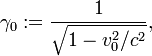$\gamma_0 := \frac{1}{\sqrt{1-v_0^2/c^2}},$
the following formulas hold:
Position: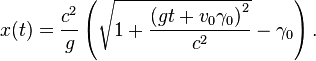$x(t) = \frac {c^2}{g} \left( \sqrt{1 + \frac{\left(gt + v_0\gamma_0\right)^2}{c^2}} -\gamma_0 \right).$
Velocity: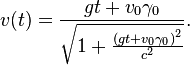$v(t) =\frac{gt + v_0\gamma_0}{\sqrt{1 + \frac{ \left(gt + v_0\gamma_0\right)^2}{c^2}}}.$
Proper time: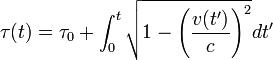$\tau(t) = \tau_0 + \int_0^t \sqrt{ 1 - \left( \frac{v(t')}{c} \right)^2 } dt'$
In the case where v(0) = v0 = 0 and τ(0) = τ0 = 0 the integral can be expressed as a logarithmic function or, equivalently, as an inverse hyperbolic function: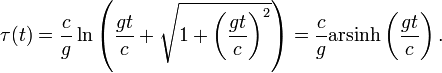$\tau(t) = \frac{c}{g} \ln \left( \frac{gt}{c} + \sqrt{ 1 + \left( \frac{gt}{c} \right)^2 } \right) = \frac{c}{g} \operatorname {arsinh} \left( \frac{gt}{c} \right) .$

### Spacetime geometry of velocity time dilation

The green dots and red dots in the animation represent spaceships. The ships of the green fleet have no velocity relative to each other, so for the clocks onboard the individual ships the same amount of time elapses relative to each other, and they can set up a procedure to maintain a synchronized standard fleet time. The ships of the "red fleet" are moving with a velocity of 0.866 of the speed of light with respect to the green fleet.
The blue dots represent pulses of light. One cycle of light-pulses between two green ships takes two seconds of "green time", one second for each leg.

As seen from the perspective of the reds, the transit time of the light pulses they exchange among each other is one second of "red time" for each leg. As seen from the perspective of the greens, the red ships' cycle of exchanging light pulses travels a diagonal path that is two light-seconds long. (As seen from the green perspective the reds travel 1.73 (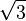$\sqrt{3}$) light-seconds of distance for every two seconds of green time.)
One of the red ships emits a light pulse towards the greens every second of red time. These pulses are received by ships of the green fleet with two-second intervals as measured in green time. Not shown in the animation is that all aspects of physics are proportionally involved. The light pulses that are emitted by the reds at a particular frequency as measured in red time are received at a lower frequency as measured by the detectors of the green fleet that measure against green time, and vice versa.

The animation cycles between the green perspective and the red perspective, to emphasize the symmetry. As there is no such thing as absolute motion in relativity (as is also the case for Newtonian mechanics), both the green and the red fleet are entitled to consider themselves motionless in their own frame of reference.
Again, it is vital to understand that the results of these interactions and calculations reflect the real state of the ships as it emerges from their situation of relative motion. It is not a mere quirk of the method of measurement or communication.